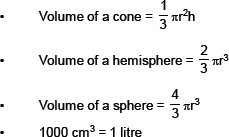# Volume and Surface Area

Volume and Surface Area: The surface area of a solid object is a measure of the total area that the surface of the object occupies. The mathematical definition of surface area in the presence of curved surfaces is considerably more involved than the definition of the arc length of one-dimensional curves, or of the surface area for polyhedra (i.e., objects with flat polygonal faces), for which the surface area is the sum of the areas of its faces.

1.    Three measures of central tendency of ungrouped data are: (i) mean, (ii) median and (iii) mode.
2.    Total surface area of a cuboid = 2[lb + bh + lh]
3.    Total surface area of a cube = 6(side)2
4.    Lateral surface area of a cuboid = Area of walls of a room = 2(l + b) × h
5.    Lateral surface area of a cube = 4a2
6.    Curved surface area of cylinder = 2πrh
7.    Total surface area of a cylinder = 2πr(r + h)
8.    Curved surface area of a cone = πrl
9.    Total surface area of a cone = πr(r + l)
10.    Surface area of a sphere = 4πr2
11.    Curved surface area of a hemisphere = 2πr2
12.    Total surface area of a hemisphere = 3πr2
13.    Volume of a’cuboid = l × b × h
14.    Volume of a cube = (side)3
15.    Volume of a cylinder = πr2rh
16.• SOLIDS AND THEIR SURFACE AREAS
The bodies occupying space are called solids, such as a cuboid, a cube, a cylinder, a cone, a sphere, etc.
These solids have plane or curved surfaces.

• SURFACE AREA OF A CUBOID AND A CUBE
The outer surface of a cuboid is made up of six rectangles. If we take the length of the cuboid as l, breadth as b and the height as h, then
Surface area of a cuboid = 2[lb + bh + hl]
Let us recall that a cuboid whose length, breadth and height are equal is called a cube. Let each edge of the cube be �a’, then
Surface area of a cube = 6a2
Note: I. Sometimes a cuboid does not have bottom and top faces. is called the lateral surface area.
II. Lateral surface area of a cuboid is 2(l + b)h.
III. Lateral surface area of a cube is 4a2.
IV. Surface area of a cuboid or cube means total surface area.